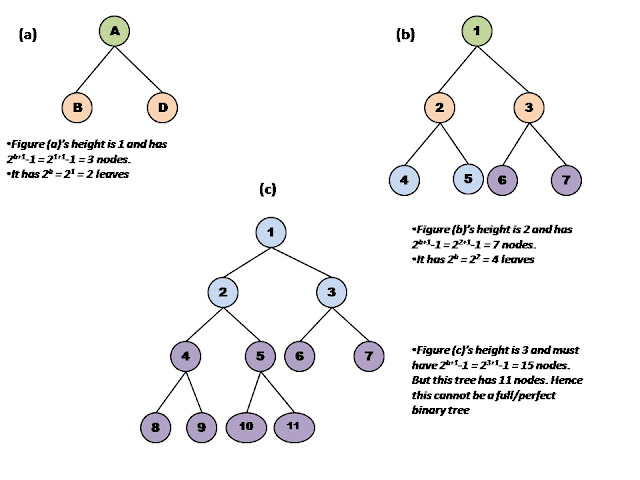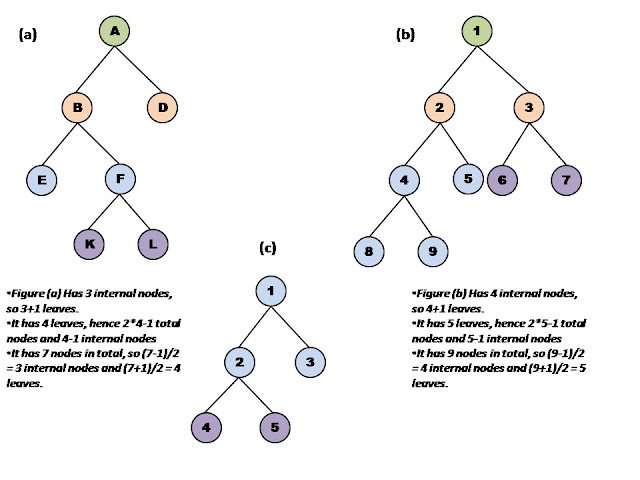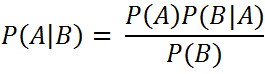`  I request GUEST WRITERS for this web site. Anyone interested please contact me at saravu2k2013@gmail.com Thanks  `
Showing posts with label Data Structures. Show all posts
Showing posts with label Data Structures. Show all posts

## Complete binary tree

A binary tree in which all the levels except the last one are completely filled with nodes and the last level is filled with nodes from left to right.

Properties:

• A complete binary tree is full and strict binary tree up to level h-1.
• A complete but non-strict binary tree with n leaves has 2n nodes (internal + external).
• A complete strict binary tree with n leaves has 2n-1 nodes (internal + external).
The following figure shows binary trees that are complete and not complete.Complete binary trees. (e) is not complete binary tree
**********

Go to Binary Tree page

complete binary tree, define complete binary tree, example of complete binary tree, how does complete binary tree differ from other types of binary trees, differentiate between complete and full binary tree

## Full binary tree

A binary tree in which every node other than the leaves has exactly two children is called full binary tree. It also referred as perfect binary tree.

Properties:

• A full binary tree of height h has exactly (2h+1 – 1) nodes (internal + external).
• A full binary tree of height h has exactly 2h leaves (external nodes).
• A full binary tree is also a strict binary tree.

The following figure shows some of the binary trees that are full binary trees;Full binary trees. (a) and (b) are full binary tree. (c) is not full binary tree
***********

Go to Binary Tree page

full binary tree, define full binary tree, example of full binary tree, how does full binary tree differ from other types of binary trees, differentiate between strict and full binary tree

## Strict binary tree

A binary tree in which every node has either 0 or two children is called strict binary tree.

Properties:

• A strict binary tree with x internal nodes has exactly x+1 leaves.
• A strict binary tree with y external (leaf) nodes has (2y – 1) nodes (internal + external) and y-1 internal nodes exactly.
• A strict binary tree with n nodes (internal + external) has exactly (n-1)/2 internal nodes and (n+1)/2 external (leaf) nodes exactly.
You can work out these properties on the example trees given below;Strict Binary Trees
**********
Go to Binary Tree page

strict binary tree, define strict binary tree, example of strict binary tree, how does strict binary tree differ from other types of binary trees, differentiate between strict and full binary tree

## Types of binary tree

• Strict Binary Tree
• A binary tree in which every node has either 0 or two children is called strict binary tree.
• Full Binary Tree
• A binary tree in which every node other than the leaves has exactly two children is called full binary tree.
• Complete Binary Tree
• A binary tree in which all the levels except the last one are completely filled with nodes and the last level is filled with nodes from left to right.

**********

Go to Binary Tree page

strict binary tree, full binary tree, complete binary tree, definition of full binary tree, example of full binary tree

### Simple introduction to Naive Bayes classifier

Simple introduction to Naive Bayes classifier What is Naive Bayes Classifier? A Naive Bayes classifier is a probabilistic classifier ...